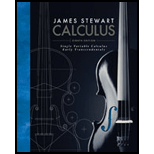Chapter 9.1, Problem 15E### Single Variable Calculus: Early Tr...

8th Edition
James Stewart
ISBN: 9781305270336

#### Solutions

Chapter
Section### Single Variable Calculus: Early Tr...

8th Edition
James Stewart
ISBN: 9781305270336
Textbook Problem

# Psychologists interested in learning theory study learning curves, A learning curve is the graph of a function P(t), the performance of someone learning a skill as a function of the training time t. The derivative dP/dt represents the rate at which performance improves. (a) When do you think P increases most rapidly? What happens to dP/dt as t increases? Explain. (b) If M is the maximum level of performance of which the learner is capable, explain why the differential equation d P d t = k ( M − P ) k   a   positive   constant is a reasonable model for learning. (c) Make a rough sketch of a possible solution of this differential equation.

(a)

To determine

To find: Time at which P increases most rapidly and to study the behavior of dPdt as t increases.

Explanation

At the beginning P increases most rapidly, it is reasonable to assume that one beginner learns more at starting time. Initially dPdt is very large

(b)

To determine

To explain: The differential equation dPdt=k(MP) is relevant model for learning.

(c)

To determine

To sketch: The possible solution of the differential equation.

### Still sussing out bartleby?

Check out a sample textbook solution.

See a sample solution

#### The Answers to Your Study Problems

Solve them all with bartleby. Boost your grades with guidance from subject experts covering thousands of textbooks. All for just \$9.99/month

Get As ASAP

## Additional Math Solutions

#### Find more solutions based on key concepts